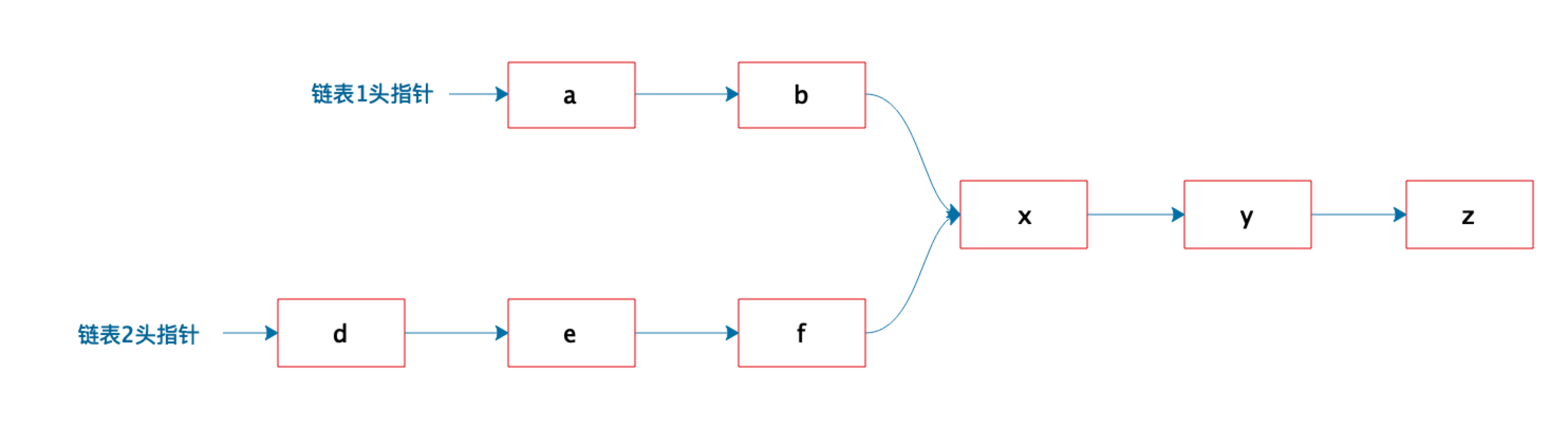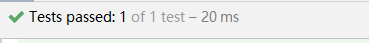# 判断两个链表是否合并Z冰红茶

﻿﻿

1、两个链表长度不同，没有重合

2、两个量表长度一样，没有重合

3、两个链表长度不同，有重合

4、两个链表长度一样，有重合

public static <T> T getMerge(Node<T> link1, Node<T> link2){        Node<T> current1 = link1;//current1先遍历链表1再遍历链表2        Node<T> current2 = link2;//current2先遍历链表2再遍历链表1        // 找到共同节点，或分别遍历完成，结束        while(!current1.value.equals(current2.value)&&(current1.next!=null && current2.next!=null)){            if(current1.next == null){                current1 = link2;            }else{                current1 = current1.next;            }            if(current2.next == null){                current2 = link1;            }else{                current2 = current2.next;            }        }        if(current1.value.equals(current2.value)){// 找到共同节点            return current1.value;        }else{// 遍历结束也没有找到共同节点            return null;        }    }    public static class Node<T>{        T value;        Node<T> next;        Node(T value){            this.value = value;        }    }

@Test    public void getMerge() {        /**         * a->b->c->x->y->z         * d->e->f->x->y->z         * 返回x         */        MergeOfLinkList.Node<String> link1 = createList(new String[]{"a","b","c","x","y","z"});        MergeOfLinkList.Node<String> link2 = createList(new String[]{"d","e","f","x","y","z"});        assertEquals("x", MergeOfLinkList.getMerge(link1, link2));        /**         * a->b->c->x->y->z         * d->e->f->g         * 返回 null         */        MergeOfLinkList.Node<String> link3 = createList(new String[]{"d","e","f","g"});        assertNull(MergeOfLinkList.getMerge(link1, link3));    }﻿## 评论